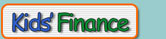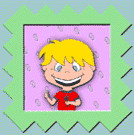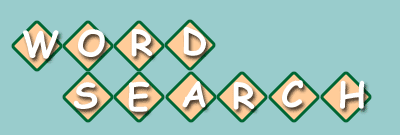### Puzzle #1 if(window.print){document.write('<form><input type=button name=print value="Print" onClick="javascript:window.print()" class="button"></form>');}

By finding the words listed below, you will add money words to your vocabulary.
Many of them are defined in the Glossary section of Money \$ense for Kids.
Look them up, then use them when you talk. Print these out and circle the words.

 R I L M O Q T P S E L L O Z M E M T L Y Z I J A T M B R R O T U I C D M N T V S T O C K L U G C A S H O C E N T N V E T R L K S V C L Q R A P D G F Y N F E X M K D O L L A R V S C M N R D P Q B U Y I L M Q Y F P F I N A N C E K H P C L L H C P S B I L L D B M T B F B K G H K S A V N U T B O N R A T P D V C I N V E S T L Z C N P Z Y L M O O F K L S Y B A K Q

Find these 16 words in the search above.

 SAVE ATM cent calculate invest dollar bill finance risk bond stock ticker return cash sell bank

| Go to Puzzle #2 |